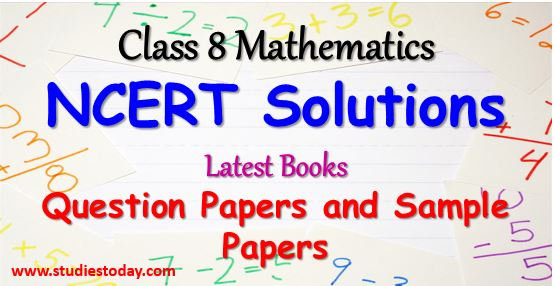# CBSE Class VIII Mathematics

Download Class 8 Mathematics NCERT Solutions for all chapters, latest sample papers and Question papers of various schools with solutions, worksheets with important questions and answers and online tests. Access free study material prepared by CBSE teachers prepared based on latest guidelines, term examination pattern and blueprint issued by CBSE and NCERT

### Constructions

Click below for Class 8 Mathematics important worksheets, latest syllabus, NCERT CBSE books, NCERT solutions, hots, multiple choice questions (mcqs), formulas, concept maps, equations, easy to learn concepts and study notes of all class 8 mathematics chapters, click on chapters below for downloading study material. Moreover , you can also Download Class 8 Social Science Study Material in PDF Form.The Class 8 Mathematics students should be able to perform the following:

1. Explore examples of rational numbers with all the operations and explore patterns in these operations.
2. Use generalised form of numbers up to 3 digits and uses her understanding of algebra to derive the divisibility rules for 2, 3, 4…. done earlier by observing patterns on them.
3. Explore patterns in square numbers, square roots, cubes and cube roots of numbers and form rules for exponents as integer.
4. Provide situations that lead to simple equations and encourage them to solve using suitable processes.
5. Experiences of multiplying two algebraic expressions and different polynomials may be provided based on their previous knowledge of distributive property of numbers and generalise various algebraic identities using concrete examples.
6. Based on their previous knowledge of factorising two numbers introduce the factorisation of algebraic expressions using relevant activities. Also do Worksheets for Class 8 Mathematics
7. Situation may be provided that involve the use of percentages in contexts like discount, profit & loss, VAT, simple and compound interest, etc.
8. Provide various situations to generalise the formula of compound interests through repeated use of simple interest.
9. A number of situations may be given where one quantity depends on the other. Encourage children to identify situations in which both the quantities increase together or in which while one increases the other decreases. For example, as the speed of a vehicle increases the time taken by it to cover the distance decreases.
10. Measure the angles and sides of different quadrilaterals and let them identify patterns in the relationship among them. Let them make their hypothesis on the basis of the generalisation of the patterns and later on verify through examples.
11. Verify the properties of parallelograms and apply reasoning by doing activities such as constructing parallelograms, drawing their diagonals and measuring their sides and angles.
12. Express/represent a 3-D shape into its 2-D form from their daily life like, drawing a box on a plane surface, showing bottles on paper, board or wall etc.
13. Make nets of various shapes like cuboids, cubes, pyramids, prisms, etc. From nets let them make the shapes and establish relationship among vertices, edges and surfaces.
14. Demonstrate the construction of various quadrilaterals using geometric kit. Also try doing NCERT Solutions for Class 8 Mathematics
15. Sketch the figure of trapezium and other polygons in the given graph paper and asked student to estimate their areas using counting of unit square.
16. Derive the formula for calculating area of trapezium using the areas of triangle and rectangle (square).
17. Identify that surfaces of various 3-D objects like cubes, cuboids and cylinder.
18. Derive formulae for surface area of cubes and cuboids using the formulae for areas of rectangles, squares and circles.
19. Demonstrate to find volume of a given cube and cuboid using unit cubes.
20. Collect data, organise it into groups and represent it into bar graphs/ pie chart. Also solve Question papers for Class 8 Mathematics
21. Conduct activities related to throwing a large number of identical dice/coins together and aggregating the result of the throws to get large number of individual events and make their assumption for the future events on the basis of the above data. Observing the aggregating numbers over a large number of repeated events also help in forecasting the chances of future events.